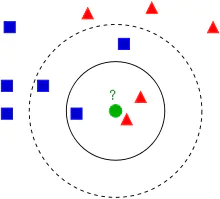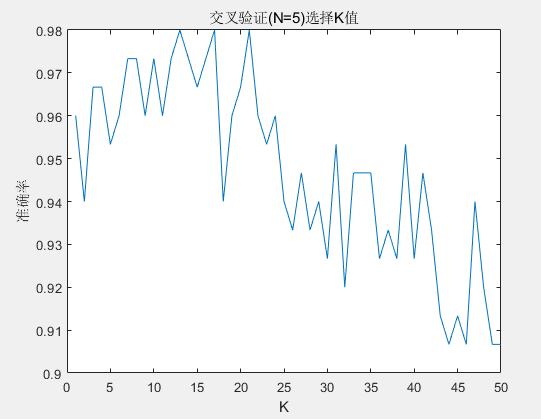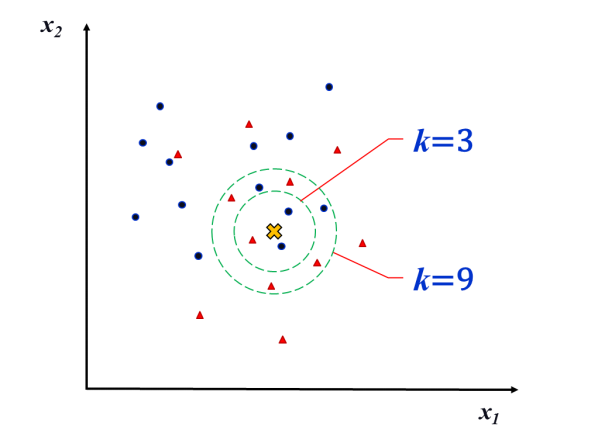• 对4组不同的信号分别采集20组，总共80组，然后经过特征提取(特征数量为8个)，得到80x8的矩阵。 划分数据集：将64组数据作为...源程序是利用KNN算法对训练集和测试集整体归一化后的测试数据进行分类，得到分类准确率。
• 模式识别KNN算法实现，基于Matlab的实现，以及剪辑近邻法的matlab实现。
• MATLABKNN算法

2021-04-20 11:40:34
【实例简介】实现KNN算法【实例截图】【核心代码】close all;clc;%%算法实现%step1、初始化训练集、测试集、K值%创建一个三维矩阵，二维表示同一类下的二维坐标点，第三维表示类别trainData1=[0 0;0.1 0.3;0.2 0.1;...

【实例简介】实现KNN算法

【实例截图】

【核心代码】close all;

clc;

%%算法实现

%step1、初始化训练集、测试集、K值

%创建一个三维矩阵，二维表示同一类下的二维坐标点，第三维表示类别

trainData1=[0 0;0.1 0.3;0.2 0.1;0.2 0.2];%第一类训练数据

trainData2=[1 0;1.1 0.3;1.2 0.1;1.2 0.2];%第二类训练数据

trainData3=[0 1;0.1 1.3;0.2 1.1;0.2 1.2];%第三类训练数据

trainData(:,:,1)=trainData1;%设置第一类测试数据

trainData(:,:,2)=trainData2;%设置第二类测试数据

trainData(:,:,3)=trainData3;%设置第三类测试数据

trainDim=size(trainData);%获取训练集的维数

testData=[1.6 0.3];%设置1个测试点

K=7;

%%分别计算测试集中各个点与每个训练集中的点的欧氏距离

%把测试点扩展成矩阵

testData_rep=repmat(testData,4,1);

%设置三个二维矩阵存放测试集与测试点的扩展矩阵的差值平方

%diff1=zero(trainDim(1),trianDim(2));

%diff2=zero(trainDim(1),trianDim(2));

%diff3=zero(trainDim(1),trianDim(2));

for i=1:trainDim(3)

diff1=(trainData(:,:,1)-testData_rep).^2;

diff2=(trainData(:,:,2)-testData_rep).^2;

diff3=(trainData(:,:,3)-testData_rep).^2;

end

%设置三个一维数组存放欧式距离

distance1=(diff1(:,1) diff1(:,2)).^0.5;

distance2=(diff2(:,1) diff2(:,2)).^0.5;

distance3=(diff3(:,1) diff3(:,2)).^0.5;

%将三个一维数组合成一个二维矩阵

temp=[distance1 distance2 distance3];

%将这个二维矩阵转换为一维数组

distance=reshape(temp,1,3*4);

%对距离进行排序

distance_sort=sort(distance);

%用一个循环寻找最小的K个距离里面那个类里出现的频率最高，并返回该类

num1=0;%第一类出现的次数

num2=0;%第二类出现的次数

num3=0;%第三类出现的次数

sum=0;%sum1,sum2,sum3的和

for i=1:K

for j=1:4

if distance1(j)==distance_sort(i)

num1=num1 1;

end

if distance2(j)==distance_sort(i)

num2=num2 1;

end

if distance3(j)==distance_sort(i)

num3=num3 1;

end

end

sum=num1 num2 num3;

if sum>=K

break;

end

end

class=[num1 num2 num3];

classname=find(class(1,:)==max(class));

fprintf('测试点(%f %f)属于第%d类',testData(1),testData(2),classname);

%%使用绘图将训练集点和测试集点绘画出来

figure(1);

hold on;

for i=1:4

plot(trainData1(i,1),trainData1(i,2),'*');

plot(trainData2(i,1),trainData2(i,2),'o');

plot(trainData3(i,1),trainData3(i,2),'>');

end

plot(testData(1),testData(2),'x');

text(0.1,0.1,'第一类');

text(1.1,0.1,'第二类');

text(0.1,1,'第三类');

标签： 算法

展开全文• knn算法matlab实现

热门讨论 2011-11-15 10:06:57
knn算法matlab实现，较简单，大家多指导指导
• 基于KNN 算法的分类器matlab实现，简单操作，可出图，可根据自身需求修改代码
• Matlab 自带KNN算法函数knnclassify实现
• Matlab实现了KNN算法中最近邻元素的查找，算出的结果与Spss完全一致KNN Matlab
• 邻近算法，或者说K最近邻（KNN，K-NearestNeighbor）分类算法是数据挖掘分类技术最简单的方法之一。所谓K最近邻，就是K个最近的邻居的意思，说的是每个样本都可以用它最接近的K个邻近值来代表。近邻算法就是将数据...
• 直接使用KNN，将自己的数据集直接代入到KNN中，可以分类出自己想要的结果
• 基于matlab采用KNN算法手写体数字识别实现 一、前言 KNN 全称是 K- Nearest Neighbors ，K-近邻。简单来说，K就是邻居个数，选出和测试样本最像的邻居（这里是欧式几何距离最短的K个邻居），那么样本的邻居是什么，...

基于matlab采用KNN算法手写体数字识别实现

一、前言

• KNN 全称是 K- Nearest Neighbors ，K-近邻。简单来说，K就是邻居个数，选出和测试样本最像的邻居（这里是欧式几何距离最短的K个邻居），那么样本的邻居是什么，样本就是什么（在K个邻居里，假如邻居的标签最多的是数字1，我们就认为样本的标签就很可能是数字1）
• KNN 实现手写体识别的原理和代码都比较简单，但网上相关文章不多，本文只是把我自己的理解写下来作为学习matlab的实践，多有纰漏，请多指教

二、实现过程

1. 处理 MNIST 数据集

• 下载 MNIST 数据集，下载测试集、测试标签、训练样本、训练标签共四个文件
• 下载下来的数据集是 IDX 文件格式的，因此用 Python 转为 50×50 的PNG图片，代码在后
• 选取合适数量的测试集和训练集，训练集中每个数字的训练样本数要一致
2. matlab 实现步骤（以图像分辨率为 50×50例）

• 对所有图片做二值化处理：有值取1，无值取0

• 将 0-9 数字的训练样本矩阵化，每一幅数字图像都是一维矩阵。以50×50分辨率图像为例，获得 1×2500 的一维矩阵；每个数字860张图片，我们就得到了 8600 × 2500 的矩阵，这作为训练矩阵

• 在训练矩阵加入标签列，用来判断某一行指的数字是多少

• 对每一幅待识别数字图像，同样将其转为 1 × 2500 的一维矩阵，称为测试矩阵

• 计算测试矩阵与训练矩阵每一维的欧氏几何距离，同样按列向量加到训练矩阵，并按距离升序按行排列训练矩阵

• 对前 K 个行向量求标签的众数，结果标签就是采用 KNN 算法得到的最有可能的识别结果

三、代码实现

1. 处理MINIST数据集的Python代码　感谢 name_s_Jimmy 的文章 使用Python将MNIST数据集转化为图片

import numpy as np
import struct

from PIL import Image
import os

data_file =  #需要修改的路径，测试或训练样本图像，如t10k-images.idx3-ubyte或train-images.idx3-ubyte
# It's 47040016B, but we should set to 47040000B
data_file_size = 47040016
data_file_size = str(data_file_size - 16) + 'B'

magic, numImages, numRows, numColumns = struct.unpack_from(
'>IIII', data_buf, 0)
datas = struct.unpack_from(
'>' + data_file_size, data_buf, struct.calcsize('>IIII'))
datas = np.array(datas).astype(np.uint8).reshape(
numImages, 1, numRows, numColumns)

label_file =  #需要修改的路径，测试或训练样本标签，如t10k-labels.idx1-ubyte或train-labels.idx1-ubyte

# It's 60008B, but we should set to 60000B
label_file_size = 60008
label_file_size = str(label_file_size - 8) + 'B'

magic, numLabels = struct.unpack_from('>II', label_buf, 0)
labels = struct.unpack_from(
'>' + label_file_size, label_buf, struct.calcsize('>II'))
labels = np.array(labels).astype(np.int64)

datas_root = r'C:\Users\TITAN\Desktop\KNN\test' #需要修改的路径
if not os.path.exists(datas_root):
os.mkdir(datas_root)

for i in range(10):
file_name = datas_root + os.sep + str(i)
if not os.path.exists(file_name):
os.mkdir(file_name)

for ii in range(10000):# 生成10000张测试或训练样本
img = Image.fromarray(datas[ii, 0, 0:50, 0:50])
label = labels[ii]
file_name = datas_root + os.sep + str(label) + os.sep + \
'mnist_train_' + str(ii) + '.png'
img.save(file_name)

print('Finished!')

1. Matlab 代码

clc;
clear;

matrix = [];% 训练矩阵
for delta = 0:9%构建训练区样本的矩阵
label_path = strcat('C:\Users\ABC\Desktop\KNN\trian\',int2str(delta),'\');
disp(length(dir([label_path '*.png'])));
for i = 1:length(dir([label_path '*.png']))
%imshow(im);
im = imbinarize(im);%图像二值化
temp = [];
for j = 1:size(im,1)% 训练图像行向量化
temp = [temp,im(j,:)];
end
matrix = [matrix;temp];
end
end

label = [];%在标签矩阵后添加标签列向量
for i = 0:9
tem = ones(length(dir([label_path '*.png'])),1) * i;
label = [label;tem];
end
matrix = horzcat(matrix,label);%带标签列的训练矩阵

%测试对象向量
for delta = 0:9%构建测试图像的向量
test_path = strcat('C:\Users\ABC\Desktop\KNN\test\',int2str(delta),'\');
len = (length(dir([test_path '*.png'])));
disp(len);
p = 0;% 识别结果计数
for i = 1:len
vec = []; %　测试样本行向量化
imshow(test_im);
test_im = imbinarize(test_im);
for j = 1:size(test_im,1)
vec = [vec,test_im(j,:)];
end

dis = [];
for count = 1:length(dir([label_path '*.png'])) * 10
row = matrix(count,1:end-1);% 不带标签的训练矩阵每一行向量
distance = norm(row(1,:)-vec(1,:));% 求欧氏几何距离
dis = [dis;distance(1,1)];% 距离列向量
end
test_matrix = horzcat(matrix,dis);% 加入表示距离的列向量

%排序
test_matrix = sortrows(test_matrix,size(test_matrix,2));
%输入K值，前K个行向量标签的众数作为结果输出
K = 5;
result = mode(test_matrix(1:K,end-1));
disp(strcat('图像',int2str(delta),'_',int2str(i),'.png','的识别结果是：',int2str(result)));

if(delta == result)
p = p + 1;
end

end
pi = p/len;
disp(strcat('识别精度为：',num2str(pi)));
disp('Finished!');
end

四、结果

• 采用 KNN (最近邻) 算法实现手写数字体的识别，经测试在 K = 5，训练样本 8600 的 条件下，总体精度在0.9以上，个别数字比如 8 识别就比较差只有 0.8 左右
• KNN 算法简单，但缺点也比较明显，运行时间长，容易收敛于局部值，精度不高。
• 提高训练样本数量，调整K值，在执行算法前对图像做初步处理可能会有更好的表现

展开全文matlab 最近邻分类算法 图像识别
• 【人脸识别】基于HOG特征KNN算法实现人脸识别matlab源码.md
• KNN算法Matlab实现 简介： KNN全称K-Nearest Neighbors,源于20世纪70年代。属于有监督学习的传统机器学习算法，既可以解决回归问题也可解决分类问题。

KNN算法Matlab实现

简介：

KNN全称K-Nearest Neighbors,源于20世纪70年代。属于有监督学习的传统机器学习算法，既可以解决回归问题也可解决分类问题。本文章只研究K-NN的分类问题，其核心思想是离样本A距离最近的K个样本的类别代表了A的类别。放一个经典案例图：在这个问题中，需要考虑三个核心要素：1）K值如何得到 2）样本间的距离如何定义 3）投票机制。

K值如何得到

答案是K折交叉验证法，此算法就是任意将训练数据集切分为K份，（K-1）份作为训练数据集，1份用于性能评估，其常被用到机器学习模型超参数调优上，此不详细赘述。

样本间的距离如何定义

包括但不限于如下：

• 曼哈顿距离
d 12 = ∑ k = 1 n ∣ x 1 k − x 2 k ∣ d_{12}=\sum^n_{k=1}|x_{1k}-x_{2k}|
• 欧几里得距离
d 12 = ∑ k = 1 n ∣ x 1 k − x 2 k ∣ d_{12}=\sqrt{\sum^n_{k=1}|x_{1k}-x_{2k}|}
• 马氏距离
D ( X i , X j ) = ( X i − X j ) T S − 1 ( X i − X j ) D(X_i,X_j)=\sqrt{(X_i-X_j)^TS^{-1}(X_i-X_j)}
• 闵可夫斯基距离
d 12 = ∑ k = 1 n ∣ x 1 k − x 2 k ∣ p p d_{12}=\sqrt[p]{\sum^n_{k=1}|x_{1k}-x_{2k}|^p}
最常用的是欧氏距离

投票机制

1. 少数服从多数
2. 距离加权投票（最常用）

代码实现

选用iris数据集作为训练测试的数据集，距离使用欧氏距离，投票机制使用少数服从多数。

iris=iris(:,2:6);
K_Max=50;
acc_avg_history=[];
for k=1:K_Max
%K交叉验证
N=5;%K交叉验证的份数
rawrank=randperm(size(iris,1));%打乱顺序
rand_iris=iris(rawrank,:);
cell_iris=mat2cell(rand_iris,150/N*ones(1,N));%分为N份
for n=1:N
cell_iris=circshift(cell_iris,1);%移位一次
train_set=cell2mat(cell_iris(1:N-1));%取N-1份做训练集
test_set=cell_iris{N};%取N份做测试集
pre_label=knn(test_set(:,1:4),train_set,k);%KNN预测花卉种类
accuracy(n)=sum(pre_label==test_set(:,5))/(150/N);%求正确率
end
acc_average=mean(accuracy);%求平均正确率
acc_avg_history=[acc_avg_history acc_average];%保存不同K值下的正确率
end
plot(acc_avg_history);xlabel('K');ylabel('准确率');title('交叉验证(N=5)选择K值')
function out=knn(test_set,train_set,K)
[n ,~]=size(test_set);
[m,~]=size(train_set);
for i=1:n
for j=1:m
%求距离
distance(j)=sqrt(sum((test_set(i,:)-train_set(j,1:4)).^2));
end
[~,index]=sort(distance,'ascend');
label=train_set(index,5);%按照距离大小排序
out(i)=mode(label(1:K));%取前K个标签里出现次数最多的那个标签
end
out=out';
end

运行结果：不同K值对应的准确率机器学习观止核心原理与实践，林学森著

展开全文算法 matlab 机器学习
• MATLAB实现KNN算法（MNIST数据集） MOOC地址：人工智能之模式识别 k-近邻分类器又称为 KNN 算法（K Nearest Neighbors Algorithm），它是指需要从训练集中找出待识别样本的 k 个最近邻，然后依据这 k 个最近邻分别...

基于MOOC人工智能之模式识别的课程完成的第五次作业
MATLAB实现KNN算法（MNIST数据集）
MOOC地址：人工智能之模式识别
k-近邻分类器又称为 KNN 算法（K Nearest Neighbors Algorithm），它是指需要从训练集中找出待识别样本的 k 个最近邻，然后依据这 k 个最近邻分别所属的类别来决定应当把待识别样本划分到哪个类别中。k-近邻分类器原理简单，无需对样本集进行回归分析或者概率分布统计，实现起来十分方便。
距离度量、k 值的选择及分类决策规则是 k 近邻算法的三个基本要素。
根据选择的距离度量（如曼哈顿距离或欧氏距离，Minkowski 明可夫斯基），可计算测试样本与训练集中的每个实例点的距离，根据 k 值选择 k 个最近邻点，最后根据分类决策规则将测试实例分类。
KNN.m 程序代码主要实现了数据集读入，KNN 分类器训练，测试及结果输出几个部分。数据集仍使用之前的MNIST数据集。

%knn.m
clc
clear variables

% 选取数据容量
train_num = 2000;
test_num = 200;
data_train = mat2vector(train_images(:,:,1:train_num),train_num);% 转换为行向量
data_test = mat2vector(test_images(:,:,1:test_num),test_num);
% 参数说明：knn要求在一个范围内选取近邻样本，这个容量需要手动给出
% 其他参数如距离计算模式，距离权重均为默认，如欧式距离，等权值等。
% 具体结果可以在运行后查看knn_model来详细观察
knn_model = fitcknn(data_train,train_labels1(1:train_num),'NumNeighbors',10);
result = predict(knn_model,data_test);
acc = 0.;
for i = 1:test_num
if result(i)==test_labels1(i)
acc = acc+1;
end
end
fprintf('精确度为：%5.2f%%\n',(acc/test_num)*100);

k 值的选择会对 k 近邻法的结果产生重大影响。在应用中，k 值一般取一个比较小的数值，通常采用交叉验证法来选取最优的 k 值。我们可以看一下 K 值的选择如何影响识别精度。展开全文模式识别 机器学习
• KNN算法及其MATLAB代码

千次阅读 2020-09-16 14:47:04
一、KNN算法原理 1.算法概述 k近邻(k-Nearest Neighbor，简称kNN)学习是一种常用的监督学习方法，其工作机制非常简单：给定测试样本，基于某种距离度量找出训练集中与其最靠近的k个训练样本，然后基于这k个"邻居...机器学习 算法
• 很有用 可运行 KNN学习算法实现，演示回归算法算法在计算机视觉的应用 ，实现如何利用偏最小二乘回归模型实现数据拟合
• KNN算法PDF，包含讲解分析，以及算法代码和结果等。对运行结果和算法进行了详细分析讲解。
• 计算方法作业要求，使用knn算法，实现对手写数字的识别，并对比在L1，L2和L∞三种范数(范数我的前面博文讲过)下的对某一个手写数字图片的识别率。 在得到题目前，一脸懵，毕竟之前没有怎么写过这种“高逼格”的程序...
• 如果机器学习能够自动识别癌细胞，那么它将为医疗系统提供相当大的益处。自动化的过程很有可能提高...从带有异常乳腺肿块的女性身上的活检细胞的测度数据入手，应用 kNN 算法，从而研究机器学习用于检测癌症的功效。
• KNN(K-Nearest Neighbor)算法Matlab实现

万次阅读 多人点赞 2017-04-16 13:30:44
KNN（K-Nearest Neighbor）算法即K最邻近算法，是实现分类器比较简单易懂的一种分类算法。K临近之所以简单是因为它比较符合人们直观感受，即人们在观察事物，对事物进行分类的时候，人们最容易想到的就是谁离那一...算法 matlab 数据 测试
• 利用matlab实现KNN算法对wine数据集的分类，并对分类结果进行了识别率的计算。KNN算法
• KNN 算法实验报告 一 试验原理 K最近邻(k-NearestNeighbor KNN)分类算法是一个理论上比 较...属于这个类别KNN算法中所选择的邻居都是已经正确分类的对象 该方法在定类决策上只依据最邻近的一个或者几个样本的类别来决
• matlab机器学习之knn算法实例

千次阅读 2020-03-26 12:17:25
KNN（K-Nearest Neighbor）算法即K最邻近算法，是实现分类器比较简单易懂的一种分类算法。 本片不讲算法原理，仅说明一下 matlab中使用该算法用的函数 详细参考：matlab官方文档 构造简单模型 Mdl = fitcknn(X,Y...机器学习 算法
• 这是一个简单的实用程序，用于对给定矩阵的缺失数据进行插补。 假设缺失数据由 NaN 值表示。 它使用一个简单的用递归进行线性搜索以找到 k 最近邻。matlab
• knn算法(k-Nearest Neighbor algorithm).是一种经典的分类算法.注意,不是聚类算法.所以这种分类算法必然包括了训练过程.然而和一般性的分类算法不同,knn算法是一种懒惰算法.它并非像其他的分类算法先通过训练建立...
• 将其进行改进，代码更加简练，并使用数据进行测试： KNN.m: function [ idx ] = KNN( trainData,trainClass,testData,K ) %UNTITLED Summary of this function goes here % Detailed explanation goes here [N,M]=...
• 基于位置指纹算法实现定位 MATLAB完整程序 包含NN KNN WKNN BAYES 四种定位算法
• KNN分类算法及MATLAB程序与结果

万次阅读 热门讨论 2016-04-14 16:08:18
KNN算法的决策过程 　k-Nearest Neighbor algorithm  　右图，绿色圆要被决定赋予哪个类，是红色三角形还是蓝色四方形？如果K=3，由于红色三角形所占比例为2/3，绿色圆将被赋予红色三角形那个类，如果K=5，由于...分类识别 算法...

matlab中knn算法matlab 订阅